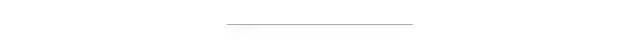# pandas 取excel 中的某一列_别找了，这是 Pandas 最详细教程了Python 是开源的，它很棒，但是也无法避免开源的一些固有问题：很多包都在做(或者在尝试做)同样的事情。如果你是 Python 新手，那么你很难知道某个特定任务的最佳包是哪个，你需要有经验的人告诉你。有一个用于数据科学的包绝对是必需的，它就是 pandas。

pandas 最有趣的地方在于里面隐藏了很多包。它是一个核心包，里面有很多其他包的功能。这点很棒，因为你只需要使用 pandas 就可以完成工作。

pandas 相当于 python 中 excel：它使用表(也就是 dataframe)，能在数据上做各种变换，但还有其他很多功能。

## pandas 最基本的功能

### 读取数据

``data = pd.read_csv( my_file.csv )data = pd.read_csv( my_file.csv , sep= ; , encoding= latin-1 , nrows=1000, skiprows=[2,5])``

sep 代表的是分隔符。如果你在使用法语数据，excel 中 csv 分隔符是「;」，因此你需要显式地指定它。编码设置为 latin-1 来读取法语字符。nrows=1000 表示读取前 1000 行数据。skiprows=[2,5] 表示你在读取文件的时候会移除第 2 行和第 5 行。

### 写数据

``data.to_csv( my_new_file.csv , index=None)``

index=None 表示将会以数据本来的样子写入。如果没有写 index=None，你会多出一个第一列，内容是 1，2，3，...，一直到最后一行。

### 检查数据

``#rows, #columns)``

``data.describe()``

### 查看数据

``data.head(3)``

``data.loc``

``data.loc[8,  column_1 ]``

``data.loc[range(4,6)]``

## pandas 的基本函数

### 逻辑运算

``data[data[ column_1 ]== french ]data[(data[ column_1 ]== french ) & (data[ year_born ]==1990)]data[(data[ column_1 ]== french ) & (data[ year_born ]==1990) & ~(data[ city ]== London )]``

``data[data[ column_1 ].isin([ french ,  english ])]``

### 基本绘图

matplotlib 包使得这项功能成为可能。正如我们在介绍中所说，它可以直接在 pandas 中使用。

``data[ column_numerical ].plot()``

().plot() 输出的示例

``data[ column_numerical ].hist()``

.hist() 输出的示例

``%matplotlib inline``

### 更新数据

``data.loc[8,  column_1 ] =  english将第八行名为 column_1 的列替换为「english」``
``data.loc[data[ column_1 ]== french ,  column_1 ] =  French``

## 中级函数

``data[ column_1 ].value_counts()``

.value_counts() 函数输出示例

### 在所有的行、列或者全数据上进行操作

``data[ column_1 ].map(len)``

len() 函数被应用在了「column_1」列中的每一个元素上

.map() 运算给一列中的每一个元素应用一个函数

``data[ column_1 ].map(len).map(lambda x: x/100).plot()``

pandas 的一个很好的功能就是链式方法(https://tomaugspurger.github.io/method-chaining)。它可以帮助你在一行中更加简单、高效地执行多个操作(.map() 和.plot())。

``data.apply(sum)``

.apply() 会给一个列应用一个函数。

.applymap() 会给表 (DataFrame) 中的所有单元应用一个函数。

### tqdm, 唯一的

``from tqdm import tqdm_notebooktqdm_notebook().pandas()``

``data[ column_1 ].progress_map(lambda x: x.count( e ))``

### 相关性和散射矩阵

``data.corr()data.corr().applymap(lambda x: int(x*100)/100)``

.corr() 会给出相关性矩阵

``pd.plotting.scatter_matrix(data, figsize=(12,8))``

## pandas 中的高级操作

### The SQL 关联

``data.merge(other_data, on=[ column_1 ,  column_2 ,  column_3 ])``

### 分组

``data.groupby( column_1 )[ column_2 ].apply(sum).reset_index()``

### 行迭代

``dictionary = {}for i,row in data.iterrows(): dictionary[row[ column_1 ]] = row[ column_2 ]``

.iterrows() 使用两个变量一起循环：行索引和行的数据 (上面的 i 和 row)

• 易用，将所有复杂、抽象的计算都隐藏在背后了；

• 直观；

• 快速，即使不是最快的也是非常快的。

• 2021编程语言「后浪」趋势预测：JavaScript、Python热度不减，但崛起最快的却是它

• 955 不加班公司名单：955.WLB

• 抖音创始人张一鸣：10 年面试 2000 人，我发现混的好的人，全都有同一个特质

• 卧槽！没想到，用Python竟能做五仁月饼

• Python 初学者进阶的九大技能

``点这里，获取新手福利``